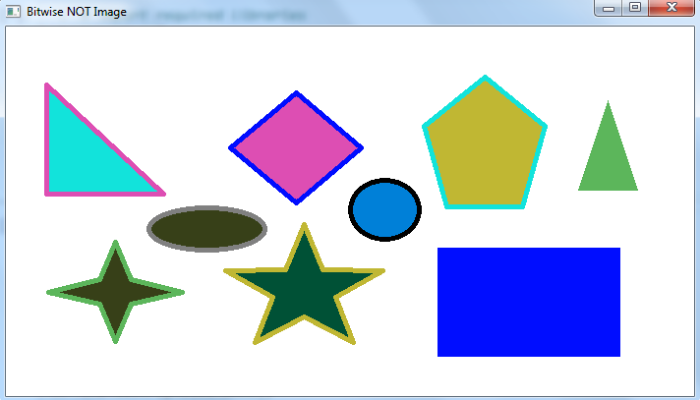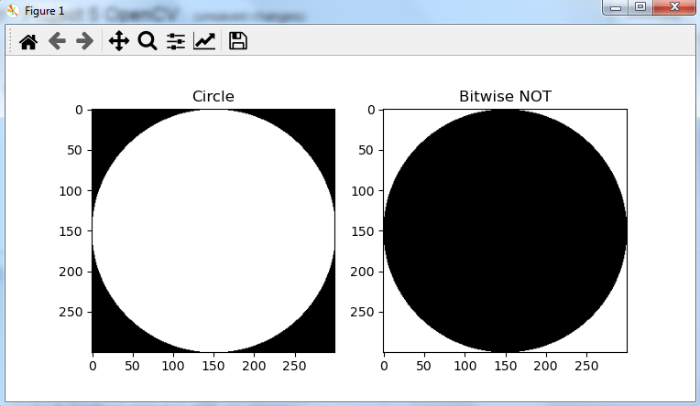# OpenCV Python â€“ How to perform bitwise NOT operation on an image?

We can perform bitwise NOT operation on an image using cv2.bitwise_not(). Here is the syntax to perform bitwise NOT operation on an image -

cv2.bitwise_not(img)


## Steps

To compute bitwise NOT on an image, you can follow the steps given below âˆ’

• Import the required library. In all the following examples, the required Python library is OpenCV. Make sure you have already installed it.

• Read the input image as a grayscale image using cv2.imread() method. Specify the full path of the image with the image type (i.e. png or jpg).

• Compute the bitwise NOT on the input image using cv2.biwise_not(img).

• Display the bitwise NOT image

Let's understand the bitwise NOT operation on an input image with the help of some Python examples.

## Example

In this example, we compute bitwise NOT of the input image.

# import required libraries
import cv2

# compute bitwise NOT on input image
not_img = cv2.bitwise_not(img)

# display the computed bitwise NOT image
cv2.imshow('Bitwise NOT Image', not_img)
cv2.waitKey(0)
cv2.destroyAllWindows()


We will use the following image as the Input File for this example âˆ’## Output

When you run the above program, it will produce the following output.Notice the color inversion of different shapes in the output window.

## Example

In this example, we compute bitwise NOT of the input image. Using this method, you can create a negative of an image.

# import required libraries
import cv2

# compute bitwise NOT on input image
not_img = cv2.bitwise_not(img)

# display the computed bitwise NOT image
cv2.imshow('Bitwise NOT Image', not_img)
cv2.waitKey(0)
cv2.destroyAllWindows()


We will use the following image as the Input File for this program âˆ’## Output

When you run the above program, it will produce the following output âˆ’Notice that the above output image is a negative image of the original input image.

## Example

In this example, we define an image circle of size 300Ã—300. We perform bitwise NOT on this image.

%matplotlib qt
# import required libraries
import cv2
import numpy as np
import matplotlib.pyplot as plt

# define an image as a circle
img = np.zeros((300, 300), dtype = "uint8")
img = cv2.circle(img, (150, 150), 150, 255, -1)

# perform bitwise NOT on image
not_img = cv2.bitwise_not(img)

# Display the bitwise NOT output image
plt.subplot(121), plt.imshow(img, 'gray'), plt.title("Circle")
plt.subplot(122), plt.imshow(not_img, 'gray'),
plt.title("Bitwise NOT")
plt.show()


## Output

When you run the above program, it will produce the following output âˆ’The above output shows the 'Bitwise NOT' image is an inverted image of the image 'Circle'.